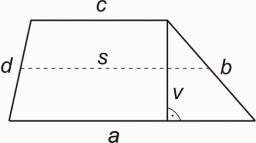# Calculate 3993

The median of the trapezoid p is 18.6 cm, and the base a = 29.8 cm. Calculate the size of the second base c.

c =  7.4 cm

### Step-by-step explanation:Did you find an error or inaccuracy? Feel free to write us. Thank you!

Tips for related online calculators
Looking for help with calculating arithmetic mean?
Looking for a statistical calculator?
Do you want to convert length units?